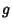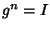Order (Group)

The number of elements in a Group, denoted. The order of an elementof a finite groupis the smallest Power ofsuch that, whereis the Identity Element. In general, finding the order of the element of a group is at least as hard as factoring (Meijer 1996). However, the problem becomes significantly easier ifand the factorization ofare known. Under these circumstances, efficient Algorithms are known (Cohen 1993).